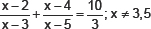# Arithmetic Progression-Sample Questions

Class X Math
1.    By increasing the list price of a book by Rs. 10, a person can buy 10 books less for Rs. 1200. Find the original list price of the book.
2.    A passenger train takes 2 hours less for a journey of 300 km, if its speed is increased by 5 km/hr from its usual speed. Find its usual speed.
3.    The numerator of a fraction is one less than its denominator. If three is added to each of the numerator and denominator, the fraction is increased by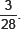Find the fraction.
4.    The difference of squares of two natural numbers is 45. The square of the smaller number is four times the larger number. Find the numbers.
5.    Solve for x: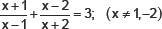6.    Using quadratic formula, solve the following for x:
9x2 – 3(a2 + b2)x + a2b2 = 0
7.    The sum of the squares of two consective odd numbers is 394. Find the numbers.
8.    Solve for x: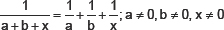9.    Find the roots of the following quadratic equation: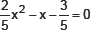10.    Find the roots of the equation: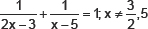11.    A natural number when subtracted from 28, becomes equal to 160 times its reciprocal. Find the number.
12.    Find two consecutive odd positive integers, sum of whose squares is 290.
13.    Find the values of k for which the quadratic equation
(k + 4)x2 + (k + 1)x + 1 = 0 has equal roots. Also find these roots.
14.    Solve for x: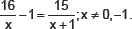15.    Solve for x: